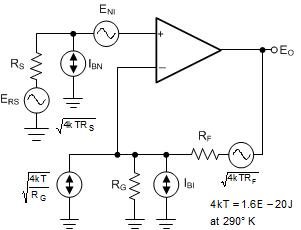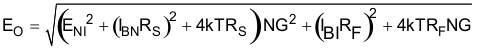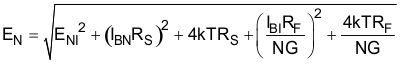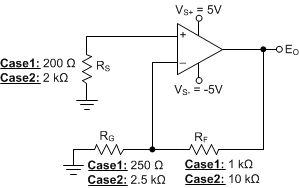JAJSFO4B August   2017  – December 2018

PRODUCTION DATA.

1. 特長
2. アプリケーション
3. 概要
4. 改訂履歴
5. Pin Configuration and Functions
6. Specifications
7. Detailed Description
1. 7.1 Overview
2. 7.2 Functional Block Diagram
3. 7.3 Feature Description
4. 7.4 Device Functional Modes
8. Application and Implementation
1. 8.1 Application Information
2. 8.2 Typical Applications
1. 8.2.1 Transimpedance Amplifier
2. 8.2.2 Multichannel Sensor Interface
9. Power Supply Recommendations
10. 10Layout
1. 10.1 Layout Guidelines
2. 10.2 Layout Example
11. 11デバイスおよびドキュメントのサポート
12. 12メカニカル、パッケージ、および注文情報

• D|8
• DCN|8
• DGK|8
• DCN|8
• DGK|8

#### 8.1.2 Noise Analysis and the Effect of Resistor Elements on Total Noise

The OPA2810 provides a low input-referred broadband noise voltage density of 6 nV/√Hz while requiring a low 3.6-mA quiescent supply current. To take full advantage of this low input noise, careful attention to the other possible noise contributors is required. Figure 72 shows the operational amplifier noise analysis model with all the noise terms included. In this model, all the noise terms are taken to be noise voltage or current density terms in nV/√Hz or pA/√Hz.Figure 72. Operational Amplifier Noise Analysis Model

The total output spot noise voltage is computed as the square root of the squared contributing terms to the output noise voltage. This computation adds all the contributing noise powers at the output by superposition, then calculates the square root to get back to a spot noise voltage. Figure 72 shows the general form for this output noise voltage using the terms shown in Equation 5.

Equation 5.Dividing this expression by the noise gain (NG = 1 + RF / RG) shows the equivalent input referred spot noise voltage at the noninverting input; see Equation 6.

Equation 6.Substituting large resistor values into Equation 6 can quickly dominate the total equivalent input referred noise. A source impedance on the noninverting input of 2-kΩ adds a Johnson voltage noise term equal to that of the amplifier (6 nV/√Hz).

Table 2 compares the noise contributions from the various terms when the OPA2810 is configured in a noninverting gain of 5V/V as Figure 73 shows. Two cases are considered where the resistor values in case 2 are 10x the resistor values in case 1. The total output noise in case 1 is 31.3 nV/√Hz while the noise in case 2 is 49.7 nV/√Hz. The large value resistors in case 2 dilute the benefits of selecting a low noise amplifier like the OPA2810. To minimize total system noise, reduce the size of the resistor values. This increases the amplifiers output load and results in a degradation of distortion performance. The increased loading increases the dynamic power consumption of the amplifier. The circuit designer must make the appropriate tradeoffs to maximize the overall performance of the amplifier to match the system requirements.Figure 73. Comparing Noise Contributors for Two Cases With the Amplifier in a Noninverting Gain of 5 V/V

### Table 2. Comparing Noise Contributions for the Circuit in Figure 73

Noise Source Output Noise Equation Case1 Case2
Noise Source Value Voltage Noise Contribution (nV/√Hz) Noise Power Contribution (nV2/Hz) Contribution (%) Noise Source Value Voltage Noise Contribution (nV/√Hz) Noise Power Contribution (nV2/Hz) Contribution (%)
Source resistor, RS ERS (1+RF/RG) 1.82 nV/√Hz 9.1 82.81 7.77 5.76 nV/√Hz 28.8 829.44 32.41
Gain resistor, RG ERG (RF/RG) 2.04 nV/√Hz 8.16 66.59 6.24 6.44 nV/√Hz 25.76 663.58 25.93
Feedback resistor, RF ERF 4.07 nV/√Hz 4.07 16.57 1.55 12.87 nV/√Hz 12.87 165.64 6.47
Amplifier voltage noise, ENI ENI (1+RF/RG) 6 nV/√Hz 30 900 84.43 6 nV/√Hz 30 900 35.17
Inverting current noise, IBI IBI (RF||RG) 5 fA/√Hz 5.0E-3 5 fA/√Hz 50E-3
Noninverting current noise, IBN IBNRS (1+RF/RG) 5 fA/√Hz 1.0E-3 5 fA/√Hz 10E-3